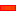﻿ Gears and Five-Bars

# Gears and Five-Bars

## Geared Five-Bar Mechanisms

Geared Five-Part mechanisms are used to give:

 • Complex coupler curves
 • Complex output motions

It is usually easier to:

 a. Join the two Parts in the dyad to the two gears.
 b. Join the two Parts in the dyad together.

####Complex Coupler CurvesGear-Pair: 1:1, Fixed-Centres, Internal Mesh

Application: Coupler Curve

In a Geared Five-bar, three Parts are the

 • Base-Part, Input Crank, and Geared-Rocker

 STEP 1:Step 1 is complete.

To remind you:

Step 2.a is complete.2.b. Add three Jointsbetween the Parts that are the Gear Pairs.

Step 2.b is complete.

Geared Five-Bar Mechanisms can give unusual motions and complex coupler curves.You may want to be more flexible with the design

 STEP 3: Edit the Part used for Gear 2

Instead of the R (Pin-Joint) at the end of the Part used for Gear 2, add a Point (with a Line) in the Part.

Use the new Point for one of the Pin-Joints in the RRR Dyad. You can edit the phase of the Gear 2 relative to Gear 1.

The design parameter options are:

 1 Gear Ratio between Gear 1 and 2 (Number-of-Teeth), Module to give centre distance
 2 Phase between the Gears
 3 Length of Gear 'Cranks'
 5 Position of Coupler PointSTEP 4: Change the number-of-teeth with the Gear-Pair dialog-box - for example 60:40.

In this case, it takes two rotations of the input crank to complete the function at the output shaft

To plot the complete Trace-Point ,you must rotate the input crank two times faster.

 STEP 5: Add a Gearing FB; make the Gear ratio = 2
 STEP 6: Connect the wire between the Linear-Motion FB, Gearing FB and the Motion-Dimension FB
 STEP 7: Connect the Output from the Motion-Dimension FB to the X input of the Graph FBHere is an interesting Coupler Curve.

In these Coupler Curves we are plotting the Point at the middle joint of the RRR Dyad.

You can add a Point to one of the Parts to give even more complex Coupler Curves.

####Geared Five-Bars as Complex Function GeneratorsGear-Pair: 1:1, Orbiting-Centres, Internal Mesh

Application: Function-Generation

Typically, you can get interesting motions from a Geared Five-bar that has a Gear-Pair with an Orbiting Centre.

The output-motion is a function of the input constant speed motion and is therefore called a Function-Generator.

 STEP 1: Add an Epicyclic Gear-PairSTEP 2: Make the gear ratio 1:1 (for example 50:50 Gear Teeth)Step 2 is complete.

 STEP 3: Add an RRR Dyadbetween the end the Geared Rocker and the Line in the Base-Part

Step 3 is complete.STEP 4: Measure the angular position of the output Part over a Machine Cycle with a Measurement FBSTEP 5: Add a Graph FBSTEP 6: Connect the Measurement FB to an input of the Graph FB

Step 6 is complete.

Add a Design-Set to give a quick way to edit the Part lengths.This Graph shows the Output Shaft Rotation as a Function of the Input, Constant Speed, Shaft Rotation.Change the Gear Ratio to give more interesting Function Generation

You can change the gear ratio of the Gear-Pair to give more complex function generation.

 STEP 7: Change the Gear ratio - for example 60:40.

In this case, it takes two rotations of the input crank to complete the function at the output shaft

 STEP 8: Add a Gearing FB; make the Gear ratio = 2
 STEP 9: Connect the wire between the Linear-Motion FB, Gearing FB and the Motion-Dimension FB
 STEP 10: Connect the Output from the Motion-Dimension FB to the X input of the Graph FBThe Graph will show the Y-axis for two rotations of the crank to give the complete Function-Generation for the 60:40 gearing ratio.

####Geared Five-Bars: Pin-Joints and Slide-JointsGear-Pair: 1:1, Fixed-Centres, Internal Mesh

Application: Coupler CurveGear-Pair, 2:1 Fixed-Centres with an RPR Dyad

Application: Coupler Curve

 • The Gear-Pair ratio changed to 60:40
 • The Crank must rotate twice for the mechanism to repeat a machine cycle.
 • To plot the complete Coupler Curve you should add a Gearing FB before the Motion-Dimension FB and make the Gearing Ratio parameter equal to 2.Application: Coupler CurveGear-Pair 1: 1, Orbiting-Centre, RPR Dyad

Application: Function-GenerationThe 'Function' at the Rocker.

It has a reasonable dwell.

Tutorial and Reference Help Files for MechDesigner and MotionDesigner 13.2 + © Machine, Mechanism, Motion and Cam Design Software by PSMotion Ltd